0

## Review: E-Z Square 6

Published on Sunday, June 30, 2013 in , , , , , , , ,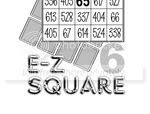It seems like Wener Miller just never stops creating!

He's just released E-Z Square 6, the latest in his series of magic square books!

E-Z Square 6 is a bit different from the previous works. Vols. 1-5 each focused on magic squares with a particular theme, such as birthdays, playing cards, and so on. What makes E-Z Square 6 different is that it goes back and updates and improves the methods and routines from past books.

The first routine is an update on the birthday magic square from E-Z Square 1. You start by putting the spectator's age in the center square of a 5 by 5 grid, and then you fill the remaining squares in a seemingly random way. When you're done, the magic total of every row, column, diangonal, and even several cross patterns, total the year the spectator was born! While the effect is the same, the method is greatly improved. Once you have the first few numbers, which is easy enough, the rest isn't much harder than counting.

The next routine is also an update on a bonus, this time on the magic square routine involving a measuring tape from E-Z Square 2. This one is a little sneakier than most of the routines, so it manages to pack an extra punch.

In E-Z Square 5, Werner Miller focused on magic squares with playing cards. The main problem with one of the feature routines, however, is that the resulting 4 by 4 squares usually featured duplicate numbers. In this volume, Werner Miller shows how to solve that problem once and for all, with a little inspiration from Richard Wiseman's The Grid, which also feature playing card magic squares.

Just when you think you've seen everything, the author goes on to teach other playing card magic square ideas with 3 by 3, 4 by 4, and 5 by 5 grids!

This ebook then rounds out with some fun magic square puzzles. One set of puzzles challenges you to cut an existing magic square into 2 smaller magic squares. The other set of puzzles require you to complete magic squares with only a few numbers with which to start. These very same puzzles, I'm proud to say, were first shared by Werner Miller to Grey Matters readers back in 2010 (puzzle 1, puzzle 2, puzzle 3, puzzle 4, answer to puzzle 4).

Technbically, you don't need the previous volumes to get use of E-Z Square 6, but reading this volume will certainly attract your curiosity about all the other routines.

If you're looking for a different take on magic squares, E-Z Square 6, which is also available in German, provides plenty of great routines and food for thought.0

## Happy Tau Day!

Published on Friday, June 28, 2013 in , , ,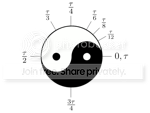Note: In honor of Tau Day, I'm re-posting and updating my original 2011 Tau Day post. Enjoy!

Happy Tau Day! Tau Day is today, but what exactly is it?

If you've been to this site before, you should be well acquainted with Pi. Like Pi (π), Tau (τ) is a a mathematical constant. The value is simple enough, in that it's 2π (2 × Pi).

Since Pi Day is 3/14, that makes Tau Day 6/28. So, why should we make such a big deal over Tau? That's the topic of today's Tau Day post!

Bob Palais started the ball rolling in 2001, with his editorial Pi Is Wrong! He wasn't talking about the actual number being a wrong quantity. When you divide a circle's circumference by it's diameter, you do get 3.1415... and so on. Palais' point was that it's the relationship to the diameter that's the basic problem.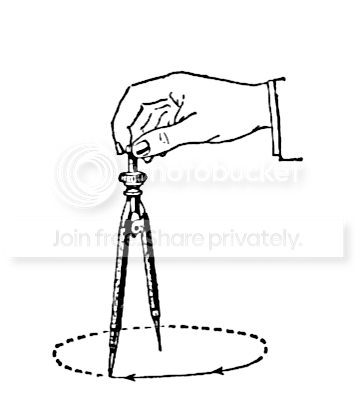Think about how you draw a circle with a compass. You place the spike at the center of your circle, and rotate the drawing implement around that point. You're taking advantage of the fact that every point on the circle is the same distance from the center of the circle.

In other words, you're taking advantage of the circle's radius, not it's diameter. Of course, if want the formula for a circle's circumference in relation to a radius (r), then you have 2πr. When you start working with the unit circle and radians (distance of the radius around the circumference), you run into 2π again, as the number of radians around a circle.

In formula after formula, 2π pops up again and again. To use Dr. C. Douglas Haessig's analogy, if Pi is a Hollywood star, then 2 is a constant groupie. Since Tau is 2 × Pi, Tau is basically the idea that these two have been dating for long enough, and it's time for them to get married.

What happens when we replace 2π with τ? I'll let Vihart, whose videos I recommended in an earlier post, explain further:

I tried to make radian conversion easy with a mnemonic (indeed, using this same video), but in the long run, you can see that Tau is a better way to go. Here are some handy links for further exploring the concepts in the above video:

Intuitive Understanding of Sine Waves
Intuitive Understanding Of Euler’s Formula
The Tau Manifesto

Robert Dixon voices his support here, and even suggests replacing Pi with τ/2:

For a better understanding of how the area is being used here, check out The Story of Pi video, and A Gentle Introduction To Learning Calculus.

Back in November 2012, Numberphile discussed the advantages of this new approach in its Tau Replaces Pi video:

Numberphile fans being passionate in the way they think about numbers, this naturally led to an intense debate just over a month later:

Ultimately, Tau may offer a better future by offering a better understanding of mathematics, and that's definitely something worthy of celebration, and thus worthy of its own day.

This only leaves one problem. Tau is twice as much as Pi, but Tau's Greek letter has only half as many legs. Just for visual consistency, shouldn't π be 2τ, and τ be .5π? Just kidding, Tau Day fans.0

## How to Win at Monopoly!

Published on Sunday, June 23, 2013 in , , ,Did you ever play the board game monopoly and wonder why others seem to make money so easily and frequently, while you could never quite the money flowing your way.

In this post, we'll use math to take a peek behind the monopoly board, and see just what strategies are the most effective for this classic game.

In Monopoly, like many board games, you move from space to space, and the only major influence on where you wind up is the space from which you started. In mathematics, processes like this are known as Markov chains. Here's a quick overview of Markov chain basics:

At first, you might think that all you'd have to do is analyze dice roll probabilities to see where you might wind up. Like any good board game, however, Monopoly throws several wrenches into the works. For example, you might roll doubles, and therefore get to roll again, and possibly even a third time. If you roll doubles a fourth time, however, you wind up in jail and everything changes!

Beyond just the dice rules, there's also the effect of the Chance and COmmunity Chest cards, which can take you to other squares on the board regardless of where you're currently located!

The analysis of the game can get complicated quick quickly. Fortunately, Walter Hickey has done all the math for you, and explains it in this straightforward slideshow over at Business Insider. The math behind each step in the thought process is clearly explained at each point, and the charts and graphs are of great help.

Take a look at this slideshow, take the time to understand it, and remember the strategies, and soon you just may find yourself dominating the next Monopoly game!0

## Mel Stover

Published on Thursday, June 20, 2013 in , , , , , ,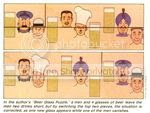Canadian Mel Stover (1912-1999) was a well-respected magician and puzzle creator whose works all seemed to have both impish and amazing qualities.

His works have been ignored far too long on the pages of Grey Matters, and I'm going to correct that today.

To give you an fun first impression of his work, read Bob Friedhoffer's remembrance of Mel Stover (PDF version). The described puzzle itself is simple enough, but when you're first confronted with it, it seems quite challenging.

Even if you're not familiar with the man himself, you've probably run across his puzzles. Puzzles.com features some of his better known puzzles, and many instantly recognize either the vanishing pencils or the matchstick giraffe right away.

As a matter of fact, Mel Stover developed numerous vanishing puzzles similar to the pencil one above. On this page, the pencils, the beer glasses, and the vanishing leprechaun were all his creations. Watch the vanishing leprechaun in the video below:

My favorite Mel Stover creation has to be what he dubbed the Beadless Abacus. As you can see below, it's an array of hexagonal cells, each containing a simple arithmetic problem. If you were challenged to sum up the answers to, say, any 3 cells in a straight line, could you do it? How about summing a line of 4 cells, or better yet, summing an array of 7 cells (1 in the center, and the 6 cells surrounding it)? Read the article below, and see if you can figure out an easy way to do these.

If you want to verify your answer, or even if you're just stumped, the answers can be found here. The feat above is an excerpt from Games Magazine's The Giant Book of Games, which is available at amazon.com, if you'd like a physical copy.

I hope you've enjoyed this look at some of Mel Stover's creations, and perhaps even found a new favorite magic trick or puzzle to share with others.0

## Still More Quick Snippets

Published on Sunday, June 16, 2013 in , , , , ,June's snippets are ready!

This month, we're going to look at computers, and especially the use of hexadecimal notation. It seems scary at first, but with a little understanding, it's easily tamed.

• Regular Grey Matters readers are very familiar with the videos from Numberphile. Here's their excellent introduction to hexadecimal, a system used with computers that help makes software and hardware engineering simpler:

• Speaking of computers and Numberphile, the makers of that channel have a newer video series, dubbed Computerphile. Not surprisingly, it's about all aspects of computers. Below is their newest release, about the early BBC B Microcomputer:

• One thing that often confuses people about hexadecimal is how to quickly recall which letters A through F go with which numbers 10-15. As long as you know that A, B, C, D, E, and F are the 1st, 2nd, 3rd, 4th, 5th, and 6th letters respectively it's actually pretty easy.

For example, what is the equivalent of 13? Simply add the two digits, 1 + 3 = 4, so it must be the 4th letter, which is D! This works for all the digits:

```10 = 1 + 0 = 1 = A
11 = 1 + 1 = 2 = B
12 = 1 + 2 = 3 = C
13 = 1 + 3 = 4 = D
14 = 1 + 4 = 5 = E
15 = 1 + 5 = 6 = F.```
Going from letters to numbers is almost as easy. If you need to recall which letter goes with which number, figure out which letter of the alphabet it is, from 1 to 6, then subtract 1 from that and put a 1 to the left of that number.

For example, what number is the equivalent of E? E is the 5th letter of the alphabet. 5 - 1 = 4, and a 1 placed to the left of that 4 makes 14. Now you can easily recall that E represents 14! With practice, these conversions will become almost automatic.

• One of the most common uses for hexadecimal in computers is to represent colors. Here are the basics of how hexadecimal numbers are used to represent colors. As with hexadecimal itself when you first learn it, it's not intuitive. Fortunately, the good people at stackoverflow.com have shared several excellent ways to develop an intuitive hexadecimal color sense.

• As mentioned in the Numberphile video above, one of the advantages of working with hexadecimal is that it converts so readily to binary when needed. To get a better understanding of this usefulness, Lazaro Diaz of the Networking Doctors shows how to work through this process:0

## Scam School Teaches the Game of 15

Published on Thursday, June 13, 2013 in , , , , , ,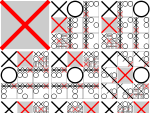Today, you'll learn an interesting new mathematical game in which the object is to obtain 3 numbers that total 15.

Well, it's not exactly new. As a matter of fact, it's something with which you are probably very familiar!

Let's jump right into the game. Watch the 274th episode of Scam School (YouTube link) below, and once they've played the game and the ad starts, stop the video and ask yourself if you can come up with any simple way to play and win the game. Once you've either figured it out or given up, go ahead and watch the remainder of the video.

Were you surprised? Yes, it's your old friend Tic-Tac-Toe (or Naughts and Crosses)! This is just one of numerous ways that have been developed to disguise the true nature this classic game. It's almost embarrassing how effective such a simple disguise can be.

Last August, I delved into strategy for the game of 15, with Part 1 teaching you the basics and how to win when you go first, and Part 2 teaching you how to win, or at least avoid losing, when you go second.

I created those posts so you can ideally play the game without ever referring to a Tic-Tac-Toe board. There's still one hitch with the game, however, and you can see it in the above video. When the game is introduced, it's explained as a mathematical game, and people immediately get apprehensive. The game is already unfamiliar, and the mathematical aspect often just adds stress.

Since you generally want to put people at ease, perhaps it's best to make the game seem more familiar. Instead of using 15 as the magic total, use 21! How would you do this? Simply increase the numbers in each part of the magic square by 2. Instead of the top row being 8, 1, and 6, you change it to 10, 3, and 8. The whole square should look like this:

```10   3   8
5   7   9
6  11   4```
Now, you can propose a game of face-up 21/blackjack, and people immediately get the idea the goal is a total of 21. It's recognizable, not some weird math game. You explain that the cards 3 through 9, a 10-value card (10, J, Q, or K) and an Ace will be laid out on the table face-up, and you and the other person will alternate taking cards, with the goal of getting exactly 3 cards that total 21.

You can even say that the Ace can be a 1 or an 11. Without the Ace, there are no combinations of 2 cards that add up to 20, so in practice it will always function as an 11 and never as a 1.

If you've learned the strategy for 15 as I teach it in my two posts linked above, there's some simple adjustments to make. Instead of 5, the center square is 7. The even numbers still represent the corners, and the odd numbers still represent the same remaining squares. The simple strategy taught by Brian in the video is also easily adaptable to the game of 21.

Try this game out, explore, and have fun with it!1

Published on Sunday, June 09, 2013 in , , ,As I write this, I'm in Vegas and it's hot. It's so brain-meltingly hot, I forgot to put up a post on Thursday!

Since the only thing my mind can conceive of is temperature right now, today's post will be about temperature conversion between Fahrenheit (°F) and Celsius (°C).

BASICS: The standard formulas taught for conversion are based on the fact that 0°C and 32°F are the same temperature. 32 is already a rough number to handle.

There's another formula that's based on the fact that -40°C and -40°F are the same temperature, and 40 is a much simpler number to deal with than 32.

To get a sense of both scales, there are some rhymes to help. For Celsius, remember: 30's warm, 20's nice, 10 is cold. 0's ice. For Fahrenheit, remember: 90's warm, 70's nice, 50's cold, 30's ice. Note that, for any temperature above -40°, Fahrenheit will be a bigger number than its equivalent in Celsius. The fact that the word Fahrenheit has a bigger number of letters than the word Celsius is an easy way to recall this.

THE FORMULAS: When converting from Fahrenheit to Celsius, follow these steps:

2) Divide by 2.

3) Multiply by 109.

4) Subtract 40.

When converting from Celsius to Fahrenheit, use these steps:

2) Multiply by 2.

3) Multiply by 910.

4) Subtract 40.

REMEMBERING THE FORMULAS: The easiest part of these formulas to remember is that, regardless of direction, the first step is always to add 40, and the last step is always to subtract 40. Already, you have half of the steps memorized!

Step 2 involves a 2, which is easy enough, but how do you remember when to multiply and when to divide? F, for Fahrenheit, is the 6th letter of the alphabet and C, for Celsius, is the 3rd letter of the alphabet. If you're going from F to C, then you can also think of that as going from 6 to 3. How do you do that? You divide by 2! Conversely, if you're going from C to F, you're going from 3 to 6, and you do that by multiplying by 2! The letters themselves practically tell you the exact step to take.

In step 3, you're always multiplying, but how do you remember whether it's 109 or 910? Remember the idea of Fahrenheit as bigger and Celsius as smaller?

When going from F to C, you're going from bigger to smaller, so the fraction should have the bigger number on top and the smaller number on the bottom: 109. When going from C to F, you're going from smaller to bigger, so the formula should have the smaller number on top and the bigger number on the bottom: 910.

Once you're able to recall the correct steps for each conversion, it's time to learn how to handle the math itself.

MENTAL MATH: The steps involving adding 40, multiplying or dividing by 2, and subtracting 40, are all easy to handle themselves. The only place you'll probably need help is mentally multiplying by the fractions.

For going from C to F, you have to multiply by 910, but how do you actually do that in your head? What 910 really means is to multiply by 9 and then divide by 10. Since 9 is equal to 3 × 3, you can break that step down farther. In short, take whatever number you have, triple it, triple it one more time, and then divide by 10. When you do that, you've effectively multiplied by 910!

Assuming you started with a whole number for C, the corresponding F temperature will either be a whole number, or will end with a single decimal place from .1 through .9, because you're only dividing by 10.

When going from F to C, you're multiplying by 109, which is the same as multiplying by 10 and then dividing by 9. Multiplying by 10 is easy enough, but you may or may not be comfortable dividing by 9. Often, you'll be dividing 3-digit numbers by 9, and some people can handle that with few problems.

For those who aren't comfortable dividing large numbers by 9, Dr. James Tanton shows you how to make this step ridiculously easy:

Notice that this method, when applied properly, also gives you the remainder. Even better, the remainder, when converted into a decimal, is just that number repeated endlessly. For example, a remainder of 8 means the number will end in .8888888..., as mentioned in the Grey Matters decimal division tutorial.

Personally, I usually give repeating decimals to 3 places, as that's enough to give people the idea.

EXAMPLES: Let's try this out with a few examples, shall we?

We'll start by converting 20°C to F. We're going from the 3rd letter to the 6th letter, which means multiplying by 2, and smaller to bigger, which means we multiply by 910, so we should already know the steps involved from memory.

1) 20 + 40 = 60

2) 60 × 2 = 120

3) 120 × 910 = (120 × 3 × 3) ÷ 10 = (360 × 3) ÷ 10 = 1080 ÷ 10 = 108

4) 108 - 40 = 68

So, if we did this correctly, 20°C should equal exactly 68°F, and double checking with Wolfram|Alpha confirms this.

How about figuring out what 87°F is in °C? We're going from the 6th letter to the 3rd letter, so step 2 is to divide by 2, and we're going from larger to smaller, so step 3 will involve multiplying by 109. Let's work through this:

1) 87 + 40 = 127

2) 127 ÷ 2 = 63.5

3) 63.5 × 109 = (63.5 × 10) ÷ 9 = 635 ÷ 9 = 70 remainder 5 = 70.555

4) 70.555 - 40 = 30.555

How close did we come to the correct answer? Wolfram|Alpha says we're exactly right!

FINAL THOUGHTS: These answers are exact, because the formulas above aren't estimates, they're the exact formulas used to convert. Many approaches taught use the idea of adding or subtracting 10% to get close, but as Numericana.com points out, adding 10% actually falls short of what needs to be added. Of course, some methods are even rougher!

Obviously, if you don't need an exact answer, the approximate methods work well. If you're going to need exact figures, however, you can have the confidence of being able to do them in your head.0

## Predicting Sums

Published on Sunday, June 02, 2013 in , , , , ,In today's post, we're going to look at a number trick presented by James Grime.

You predict the total of several 4-digit numbers, but don't worry. It's fun, simple, and straightforward.

The following routine is a quick video from the Numberphile series of videos:

The most commonly-used source for this routine is Martin Gardner's book, Mathematics, Magic & Mystery, in which it has the simple title of “Predicting A Sum”. Interestingly, the example number used in the book is 3,845, very close to James Grime's chosen example number, 3,485.

There are many ways to make this routine more impressive. You could employ a secret help, who provides the needed numbers at each step. This takes the heat off of your choices, and makes the whole routine seem fairer, and more impressive.

Another idea to consider is that you don't have to do this as a prediction. Instead, you can do it as a feat of mental arithmetic. Have two people, one of whom is your secret helper, write the numbers down out of your view, and then show the numbers to you briefly. You apparently quickly memorize and calculate the numbers in your head faster than they can do it with a calculator!

With a little creativity, you can think of numerous ways to perform this. Want to seem psychic? Divine the total without ever seeing the numbers (thanks to your secret helper again). You could also perform a routine similar to Scam School's first Pi Day Magic Trick, in which they circle a number, read off the remaining digits, and you can determine the digit or digits they didn't name. Since you know in advance what the total will be, it's all recall with no calculation!

There's a routine with a similar basis called “Alberti's Game”, in which you and two other people randomly choose 3-digit numbers to create 2 multiplication problems, and you're able to predict the sum of the answers to those multiplication problems. Because there's several steps involved, and the answers tend to be large 6-digit numbers, this version seems even more impressive.

How is it done? The answer is below, courtesy of Karl Fulves' Self-Working Number Magic (Amazon link):

Just as with the previous routine, a little creativity, and the possible secret helper, can yield some very amazing results.

Try these out, and if you like them,show your appreciation to Grey Matters by buying Martin Gardner's book, Mathematics, Magic & Mystery and/or Karl Fulves' Self-Working Number Magic. You'll find an amazing variety of number-based magic in both of these mathemagical classics!